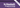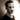ListsAh, another data type deep dive! This time we're looking at lists and how they are created and evaluated. Not much more of an introduction needed here; let's just dive right in!

## List creation

There's a number of ways to construct lists in Haskell. This chapter covers using ranges and list comprehensions, both of which are quite neat and something I get deep personal satisfaction out of (hey, I'm not weird!).

We covered ranges when looking at the Enum typeclass in Chapter 6, so let's just do a quick refresher: In Haskell you can create lists out of anything that implements the Enum typeclass (such as Int and Char and Bool) by using the syntactic range sugar:

-- expression and what they evaluate to
[ 1 .. 5 ] -- [1, 2, 3, 4, 5]
[ 1 .. ] -- an infinite list of integers, each entry increasing by 1
[ 1, 3 .. ] -- an infinite list of integers, each entry increasing by 2
[ 1, 5 .. 10 ] -- [1, 5, 9] (step size is 5-1 = 4)

Neat, huh? Just keep in mind that the range must be in increasing order. If you try something like [5 .. 1], you'll get an empty list.

In addition to ranges, there's also this thing called list comprehension. List comprehensions are something I normally associate with Python, but I think Haskell's version is much cooler. The syntax looks a lot like set comprehension does in mathematics (which is also where the concept comes from). A list comprehension requires at least one list (called a generator) that provides an input for the comprehension. Here's a simple comprehension:

[ x^2 | x <- [1 .. 5] ] -- [1, 4, 9, 16, 25]

The first bit before the pipe, x^2, is the expression that goes into every cell. The variable x is assigned from the generator [1 .. 5]. Indeed, what this list comprehension does is simply to square each number of the generator. The same result could be achieved by a simple map: map (^2) [1 .. 5].

Where list comprehensions really shine, however, is when you start to add more variables and optional filters. When adding more variables, the comprehension will create a list with every possible permutation of those values. For instance: say you want to create a list of all the positions in a 3x3 grid around an origin ((-1, -1) to (1, 1)). Using list comprehensions, that's trivial:

[ (x, y) | x <- [-1 .. 1], y <- [-1 .. 1] ]

In addition to variables, you can also add filters after the variables. Say you want the same list as above, but you don't want to include the origin ((0, 0)):

[ (x, y) | x <- [-1 .. 1], y <- [-1 .. 1], (x, y) /= (0, 0) ]

And not just one filter; you can add multiple! What if we want a bigger list, but only want the tiles where the Manhattan distance to the origin is divisible by 7 (and excluding the origin itself)?

[ (x, y) | x <- [-4 .. 4], y <- [-4 .. 4], (x, y) /= (0, 0), (abs x + abs y) mod 7 == 0 ]

It may be a farfetched example, but I think it adequately shows just how cool list comprehensions can be in Haskell.

## Spines and non-strict evaluation

To talk about list evaluation, we need to talk about how lists are represented. To talk about how lists are represented, we need to talk about spines. But let's take a step back. It's not all that scary.

The list data type is defined as such:

data [] a = [] | a : [a]

It's a recursive data structure. It's either an empty list, or an element prepended to another list. Syntactically, when we write lists or print them, they're usually presented like this: [1, 2, 3], but can also be thought of as 1 : 2 : 3 : []---that is 1 before 2 before 3 before the empty list.

The : operator is known as cons. The list construction in the last paragraph can be thought of as a series of cons cells (a value consed onto a list). It could also be written like this, which may make the relation to the list data constructor clearer: 1 : (2 : (3 : [])).

One problem with this representation, though, is that it makes it seem like 1 exists 'outside' of the list, when it is actually contained by it (and by its cons cell). Because of how lists are evaluated in Haskell, we'll see that because the value is contained, we can evaluate cons cells without evaluating their contents.

Let's talk about spines. You see, the list above can also be represented---conceptually---like this:

:
/ \
1   :
/ \
2   :
/ \
3  []

In this representation, the cons operators line up to form what is known as the spine, which is a fancy word for the connective structure that makes up a data structure such as a list or a tree. In the case of lists, it's just a series of cons operators.

Crucially, spines are evaluated independently of values. What does this mean? Well, functions that only require evaluating the spine and not the values, such as length, can walk down a list without evaluating the contents. So, returning to our list from before, if we were to apply the length function on it (and the values hadn't already been evaluated), you could think of it like this, where the underscores represent values that haven't been evaluated.

:
/ \
_   :
/ \
_   :
/ \
_  []

Another way to get this point across is to look at what happens when we add bottom values to a list. Run this in the REPL to try it out.

let xs = [1, undefined, 3]
length xs -- 3
take 1 xs -- 
take 2 xs -- Exception: Prelude.undefined

Taking the length of the list works just fine because we don't need to evaluate the items in the list. Taking the first element is no problem because it's a regular value and the rest of the list has not been evaluated. When we take two values, however, this forces the evaluation of the second item in the list, undefined, which causes the program to crash.

There is loads more to be said about list evaluation and the impacts it has on what we can and can't do in code, but these are the most important things covered in this chapter.

## Chapter definitions

• Product type ::

A product type is a type that contains several other types at the same time. The canonical example is a tuple: You can have a tuple of a Bool and an Int, and for every instance you have of that type, you will have both a Bool and an Int. The reason its called a product type is that the number of possible permutations is the product of the number of possible values for each type.

Say you have a data type: data Direction = Up | Down | Left | Right. If you have a (Bool, Direction) tuple, you now have $8$ ($4 \cdot 2$) different possible permutations of that tuple.

• Sum type ::

A sum type is a type that is only one of a set number of types at any time. Relating this back to the example above with Bool and Int: If you have a data type that is either a Bool or an Int (such as Either Bool Int), then that's a sum type. The number of possible permutations is the sum of the number of possible values for each type.

Using the Direction type from above, Either Bool Direction has $6$ ($4 + 2$) possible values.

• Cons ::

The : operator. Used to prepend a value onto a list. Can also be used as a verb (e.g. consing a value onto a list).

• Cons cell ::

A data constructor and a product of the types a and [a] as defined in the list data type definition.

• Spine ::

A structure that strings a collection of values together, the scaffolding of a data structure. For the list datatype, it's formed by the recursive nesting of cons cells.

## Other topics

In addition to what we have covered here, the chapter also contains more information about pattern matching on lists, and some common list operations: map, filter, and zip. However, to keep things nice and brief, I've decided to leave them out for now. For more information on those topics, please consult your search engine of choice or nearest message board.Thomas Heartman is a developer, writer, speaker, and one of those odd people who enjoy lifting heavy things and putting them back down again. Preferably with others. Doing his best to gain and share as much knowledge as possible.